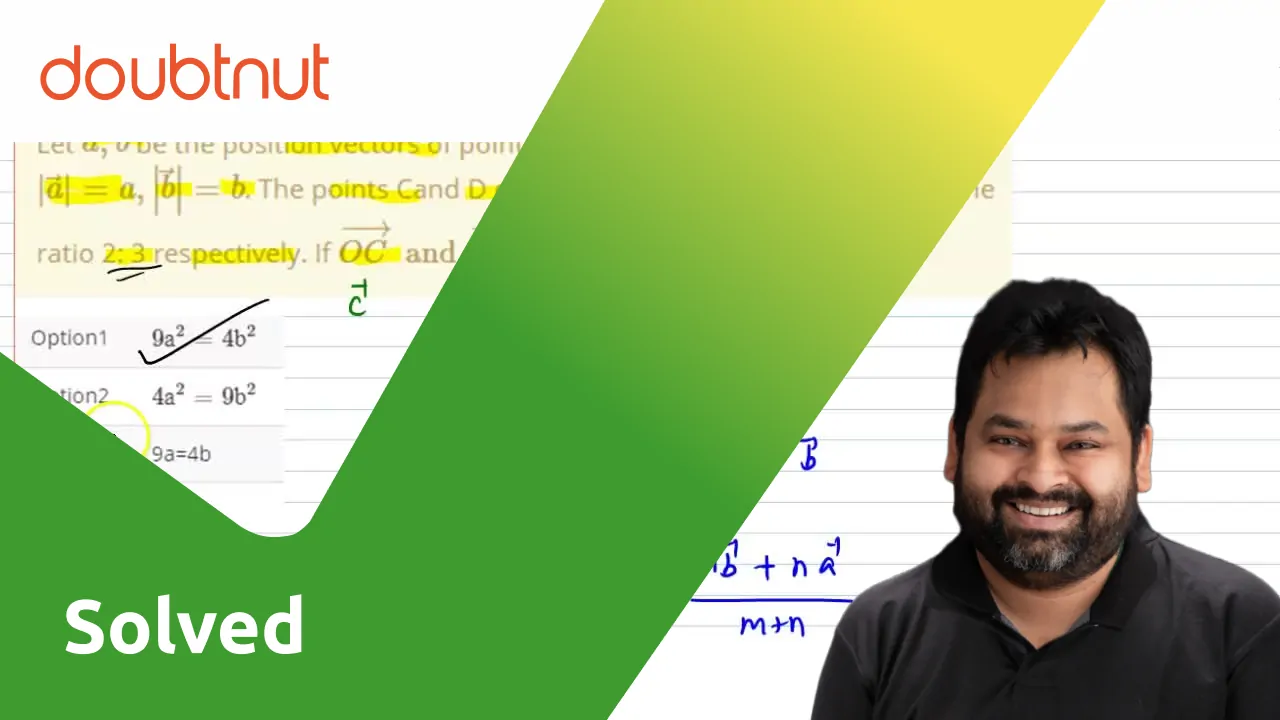Doubtnut App - Get 50 Lakh+ video solutions better than GoogleInstall Now

# Let →a,→b be the position vectors of points A and B with respect to and |→a|=a,∣∣→b∣∣=b. The points Cand D divide AB internally and externally in the ratio 2: 3 respectively. If −−→OCand−−→OD are perpendicular, then

A

9a2=4b2

B

4a2=9b2

C

9a=4b

D

4a=9b

Text Solution
Verified by Experts

Video Solution